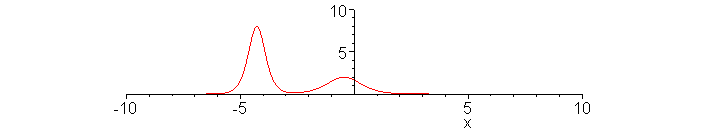### Solitons

 SolitonsWaves are in general dispersive and the original wave form becomes dispersed as waves propagate over a large distance. However, in some waves, dispersion can be compensated by nonlinearity and they can propagate over a large distance keeping original wave forms. Waves in shallow water and plasma waves known as the ion acoustic wave are typical examples. In fact both waves can be described by a common nonlinear wave equation (KdeV equation) originally derived by Kortweg and de Vries.Animation shows propagation of large (amplitude = 8) and small (amplitude = 2) amplitude solitons and their passing collision. Soliton propagates faster as its amplitude increases and if two solitons of different amplitudes are created, collision can occur. Note that collision does not destroy wave forms of either wave. Snapshots before, at, and after collision are also shown. At the instant of collision, the wave amplitude becomes smaller than the sum of the two waves. This is a typical nonlinear behavior wherein the superposition principle, which works universally in linear waves, entirely breaks down. Similar nonlinear wave propagation occurs in light waves guided along an optical fiber. Fast, larger amplitude soliton. Amplitude = 8.> with(plots):animate(8*(sech(2*(x-.5*16*t)))^2,x=-10..10,t=-1.2..1.2,frames=49,numpoints=500,color=red,view=[-10..10,0..10]);Slow, smaller amplitude soliton. Amplitude = 2. Velocity is one half of that of the fast soliton.> animate(2*(sech(x-.5*4*t))^2,x=-10..10,t=-1.2..1.2,frames=49,numpoints=500,color=red,view=[-10..10,0..10]);Passing collision of fast and slow solitons.> with(plots):animate(12*(3+4*cosh(2*x-.5*8*t)+cosh(4*x-.5*64*t))/(3*cosh(x-.5*28*t)+cosh(3*x-.5*36*t))^2,x=-10..10,t=-1.2..1.2,frames=49,numpoints=1000,color=red,view=[-10..10,0..10]);Snapshot at t = -1.> t:=-1:plot(12*(3+4*cosh(2*x-.5*8*t)+cosh(4*x-.5*64*t))/(3*cosh(x-.5*28*t)+cosh(3*x-.5*36*t))^2,x=-10..10,numpoints=1000,color=red,view=[-10..10,0..10]);Snapshot at t = -0.5.> t:=-0.5:plot(12*(3+4*cosh(2*x-.5*8*t)+cosh(4*x-.5*64*t))/(3*cosh(x-.5*28*t)+cosh(3*x-.5*36*t))^2,x=-10..10,numpoints=1000,color=red,view=[-10..10,0..10]);Snapshot at t = 0. Note that the amplitude is only 6.0. The pulse width is broadened.> t:=0:plot(12*(3+4*cosh(2*x-.5*8*t)+cosh(4*x-.5*64*t))/(3*cosh(x-.5*28*t)+cosh(3*x-.5*36*t))^2,x=-10..10,numpoints=1000,color=red,view=[-10..10,0..10]);Snapshot at t = 0.5.> t:=0.5:plot(12*(3+4*cosh(2*x-.5*8*t)+cosh(4*x-.5*64*t))/(3*cosh(x-.5*28*t)+cosh(3*x-.5*36*t))^2,x=-10..10,numpoints=1000,color=red,view=[-10..10,0..10]);Snapshot at t =1.> t:=1.0:plot(12*(3+4*cosh(2*x-.5*8*t)+cosh(4*x-.5*64*t))/(3*cosh(x-.5*28*t)+cosh(3*x-.5*36*t))^2,x=-10..10,numpoints=1000,color=red,view=[-10..10,0..10]);Ċ
Jason Lin,
2018年9月6日 凌晨12:43